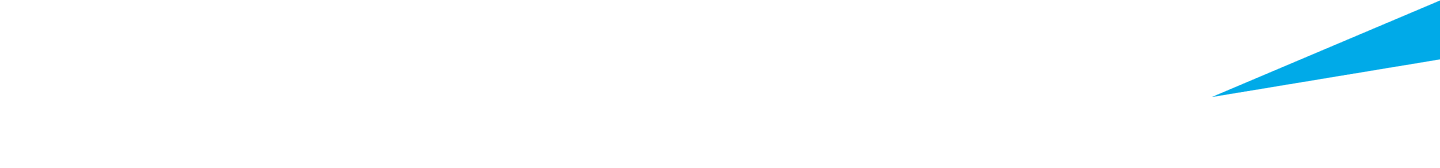# Matlab Certification Training Course

Learn the basics of MATLAB so you can start working with complicated mathematical functions and creating intriguing matrix visuals.

###### MATLAB Courses

Learn the basics of MATLAB so you can start working with complicated mathematical functions and creating intriguing matrix visuals. Learning MATLAB may lead to a variety of career opportunities in fields such as data science, data analysis, computer science, Python, Java, and more. Begin learning right now!

#### What is MATLAB?

MATLAB is a programming language developed by MathWorks that is used for numerical computing tasks including matrix manipulations, data visualizations, and advanced mathematical computations. MATLAB Simulink is a graphical programming environment which, when used together with MATLAB, allows developers and designers to model and test systems prior to production.

#### Online MATLAB Courses and Programs

Get an introduction to MATLAB online with courses from École Polytechnique Fédérale de Lausanne and the Technical University of Munich. Courses are available in English, French and German and cover MATLAB basics such as writing a MATLAB function or making a MATLAB plot diagram. The short, self-paced courses are free and will help you get up and running quickly.

Edx courses help you learn and use MATLAB programming in an engaging and effective online environment complete with video tutorials, quizzes and more. Additionally, edX offers the option to pursue verified certificates in MATLAB courses. The certificate lists edX and the name of the university or institution offering the course and can be uploaded to your LinkedIn profile. It is proof for employers and others that you have successfully completed the course.

In addition to MATLAB software and programming courses, edX offers free online courses in math, data visualization and much more. Many courses are self-paced so you can enroll and start learning today.